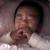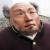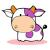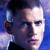php查询数据，再根据得出的结果循环查询

id  user  pwd  vid
1    a    123
2    b    345   1
3    c    567   2
4    d    789   3
5    e    012   3
6    f    345   4

```\$user=\$_POST['user'];
\$s="select * from `tabe` where `user` = '\$user'";
\$quer=mysql_query(\$s);
while(\$r=mysql_fetch_array(\$query)){
\$userid=\$r;
}

if(\$userid){
\$sql="select * from `tabe` where `vid`='\$userid'";
\$q=mysql_query(\$sql);
while(\$row=mysql_fetch_array(\$q)){
echo \$row;
}
}```

01. 貌似在做“x度人脉”？这类需求几年前社交网站盛行时候多，计算量较大，这是其数学性质决定的，不仅仅是技术问题

2. 这种需求用图数据库比较好。

3. 如果要用关系数据库，仿照“无限分类”各种思路做。

4. 可以把问题看成图中从节点a出发，获取和a连通的所有节点。

0\$userid只有一个值当然只会查询一次了。建议是不要在循环里面做查询，你可以用in子查询，这样效率会高很多
0```#先查出第一个userid
\$user=\$_POST['user'];
\$s="select id from `tabe` where `user` = '\$user'";
\$quer=mysql_query(\$s);
\$r=mysql_fetch_array(\$query)
\$userid=\$r;
#递归查询
fu(\$userid);

function fu(\$userids)
{
#先保证参数是数组
if(!is_array(\$userids))
\$userids = array(\$userids);
#把要查的id拚成一串
\$where = '("'.import('","',\$userids).'")';
#拚sql
\$sql="select id from `tabe` where `vid` in {\$where}";
\$q=mysql_query(\$sql);
#如果vid不存在
if(!mysql_row_num(\$q))
return ;
#取出所有的ID
while(\$row=mysql_fetch_array(\$q)){
echo \$row;
\$userids[] = \$row;
}
#递归
fu(\$userids);
}```

0```\$user=\$_POST['user'];
\$s="select * from `tabe` where `user` = '\$user'";
\$quer=mysql_query(\$s);
while(\$r=mysql_fetch_array(\$query)){
\$userid=\$r;
}

if(\$userid){
search(\$userid);
}

\$a = array();
function search(\$id){
global \$a;
sql = "select * from `table` where vid='\$id'";
\$query = mysql_query(\$sql);
\$rs = array();
while(\$row = mysql_fetch_array(\$query)){
\$rs[] = \$row;
}
if(!empty(\$rs)){
foreach(\$rs as \$k=>\$v){
\$a[] = \$v;
search(\$v['id']);
}
}
}
//试试。```